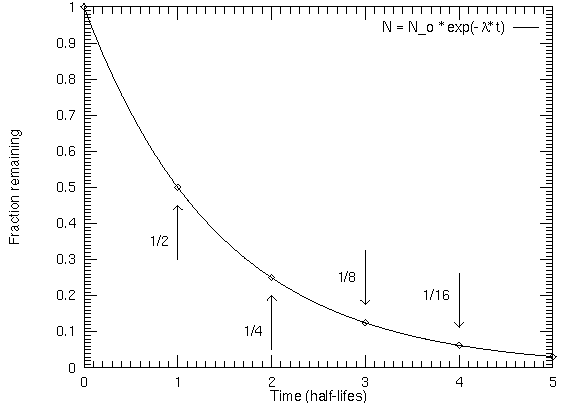# Phosporous-32 is a radioisotope used in molecular biology After 44 days, 17% of the sample remains. How do you calculate the half-life?

Dec 5, 2016

You can do it like this:

#### Explanation:

The expression for 1st order decay is:

$\textsf{{N}_{t} = {N}_{0} {e}^{- \lambda t}}$

$\textsf{\lambda}$ is the decay constant.

The graph looks like this:The key to the problem is to find the decay constant $\textsf{\lambda}$ as this is directly related to the 1/2 life.

Taking natural logs of both sides gives:

$\textsf{\ln {N}_{t} = \ln {N}_{0} - \lambda t}$

$\textsf{\ln 17 = \ln 100 - l a m \mathrm{da} \times 44}$
$\therefore$$\textsf{2.833 = 4.605 - l a m \mathrm{da} \times 44}$
$\therefore$$\textsf{\lambda = \frac{1.772}{44} = 0.0403 \textcolor{w h i t e}{x} {d}^{- 1}}$
$\textsf{{t}_{\frac{1}{2}} = \frac{0.693}{\lambda} = \frac{0.693}{0.0403} = 17 \textcolor{w h i t e}{x} d}$